# 微积分符号

## 微积分和分析数学符号表限制 函数的极限值
ε ε 代表一个非常小的数字，接近零 ε 0
e e常数/欧拉数 e = 2.718281828 ... e = lim（1 + 1 / xxx →∞
ÿ 衍生物 导数-拉格朗日符号 （3 x 3）'= 9 x 2
y '' 二阶导数 导数的导数 （3 x 3）''= 18 x
y n n阶导数 n次推导 （3 x 3（3） = 18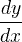衍生物 导数-莱布尼兹的符号 d（3 x 3）/ dx = 9 x 2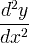二阶导数 导数的导数 d 2（3 x 3）/ dx 2 = 18 xn阶导数 n次推导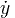时间导数 时间导数-牛顿符号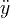时间二阶导数 导数的导数
d X ÿ 衍生物 导数-欧拉符号
d X 2 ÿ 二阶导数 导数的导数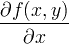偏导数   ∂（X 2 + ý 2）/∂ X = 2 X

[ ab ] 封闭间隔 [ ab ] = { x | 一个Xb }
ab 开放间隔 ab）= { x | a < x < b }

z * 复合共轭 z = a + biz * = a - bi z * = 3 + 2
z 复合共轭 z = a + biz = a - bi z = 3 + 2
Re（z 复数的实部 z = a + bi →Re（z）= a Re（3-2 i）= 3
Im（z 复数的虚部 z = a + bi →Im（z）= b Im（3-2 i）= -2
| z | 复数的绝对值/幅值 | z | = | a + bi | =√（a 2 + b 2 | 3-2 i | =√13
arg（z 复数的论点 复杂平面中的半径角 arg（3 + 2 i）= 33.7°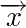向量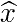单位向量
x * y 卷积 yt）= xt）* ht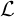拉普拉斯变换 Fs）={ ft）}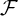傅里叶变换 Xω）={ ft）}
δ 三角函数
lemniscate 无限符号